# Test Prep Plan - Take a practice test

Take this practice test to check your existing knowledge of the course material. We'll review your answers and create a Test Prep Plan for you based on your results.
How Test Prep Plans work
1
2Based on your results, we'll create a customized Test Prep Plan just for you!
3Study smarter
Study more effectively: skip concepts you already know and focus on what you still need to learn.

# Geometric Shapes & Properties Chapter Exam

Exam Instructions:

Choose your answers to the questions and click 'Next' to see the next set of questions. You can skip questions if you would like and come back to them later with the yellow "Go To First Skipped Question" button. When you have completed the practice exam, a green submit button will appear. Click it to see your results. Good luck!

### Page 1

#### Question 1 1. What is the perimeter of the pictured square?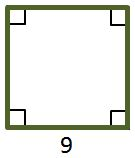#### Question 2 2. What is the area of the right triangle shown below?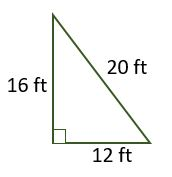#### Question 4 4. If angle 1 measures 70, what does angle 3 measure?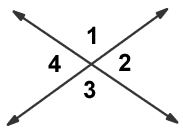### Page 2

#### Question 6 6. In the picture shown below, there are three lines marking each side of the triangle. These lines give the indication that the triangle is: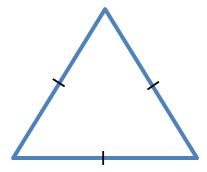#### Question 7 7. Mary claims that the triangles below are similar by AA. Do you agree or disagree with Mary?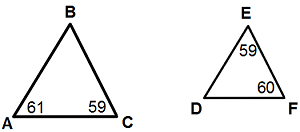#### Question 8 8. What is shown below?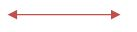### Page 3

#### Question 11 11. Study the given diagram. Find the value of x, the distance between T and E.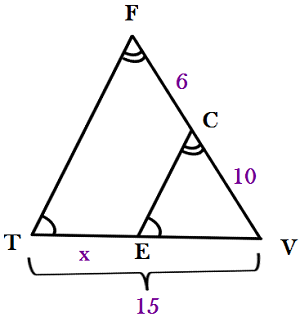#### Question 12 12. What is the area of the pictured triangle?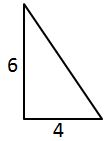#### Question 13 13. What shape is the candle in this candle holder?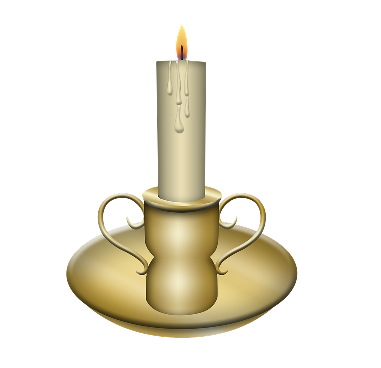#### Question 14 14. The isosceles triangle pictured below shows one angle measuring 40 degrees. What is the measure of angle x?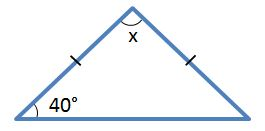### Page 4

#### Question 16 16. In the figure below, Angle 7 = 15x and Angle 10 = 9x + 30. Determine the measure of Angle 10.#### Question 17 17. Josh claims that these triangles are similar by SSS. Do you agree or disagree with Josh?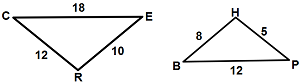#### Question 18 18. The pictured shape is a: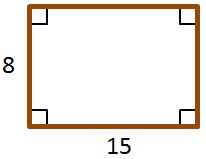### Page 5

#### Question 22 22. In triangle GBN, Angle G is seven more than three times a number, Angle B is five more than four times the number, and Angle N is twelve less than five times the number. Solve for the measure of Angle G.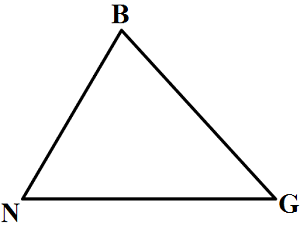#### Question 23 23. What is the area of the annulus/doughnut shown below?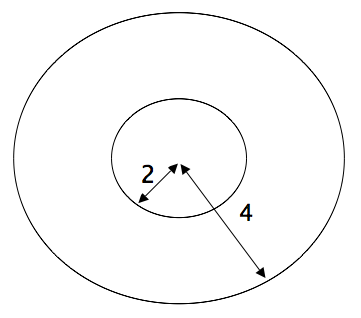### Page 6

#### Question 28 28. What is the relationship between Angle 1 and Angle 7?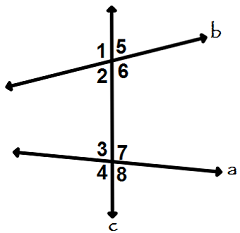#### Question 29 29. Given the figure below, Angle 3 = 140y and Angle 5 = 80y + 70. Determine the value of y.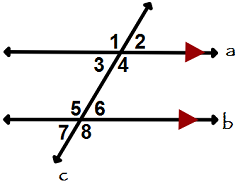#### Geometric Shapes & Properties Chapter Exam Instructions

Choose your answers to the questions and click 'Next' to see the next set of questions. You can skip questions if you would like and come back to them later with the yellow "Go To First Skipped Question" button. When you have completed the practice exam, a green submit button will appear. Click it to see your results. Good luck!

Support